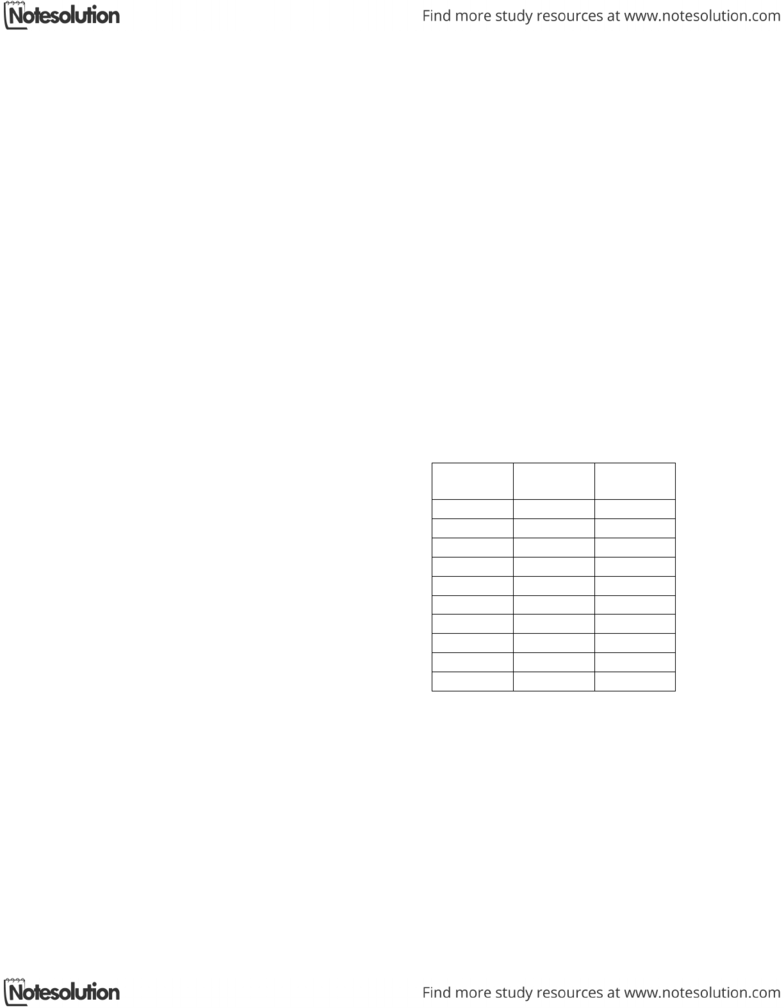# ECE385H1 Study Guide - University Of Toronto Faculty Of Applied Science And Engineering, Endianness, Assembly Language

53 views11 pages
Page:
of 11Page 1 of 11 Last Name (in case pages get detached):__________________
UNIVERSITY OF TORONTO
FACULTY OF APPLIED SCIENCE AND ENGINEERING
MIDTERM EXAMINATION, MARCH 2007
ECE243H1 S – COMPUTER ORGANIZATION
Exam Type: D
Duration: 2 Hours
A. Moshovos and G. Steffan
This is a type D exam. You are allowed to use any
printed material but not a calculator.
Last Name (Print): __________________
First Name: _________________________
Student Number: _____________________
Marks
Max.
Marks
1 20
2 10
3 10
4 10
5 10
6 10
7 10
8 10
9 10
Total 100
Use your time wisely as not all questions will require the
same amount of time. If you think that assumptions must be
multiple possibilities, comment that there are, explain why
and then provide at least one possible answer and state the
corresponding assumptions.
Page 2 of 11 Last Name (in case pages get detached):__________________
Marking starts at the maximum grade per question and you deduct as per the
instructions. If you reach 0 you stop.
Page 3 of 11 Last Name (in case pages get detached):__________________
1.  Basic Instruction Semantics: Assume the following initial state for
registers:
R8 = 0x12345678
R9 = 0xFAFB12AE
R10 = 0x01000100
R11 = 0xFFFFFFFE
R12 = 0xFFFFFFFF
In the following lists of instructions, determine what is the outcome of the instruction and
write in hexadecimal using all eight digits (that is write 0x00000000 and not 0x0). In all
cases find the outcome of each instruction given that it executes with the initial state
given above.
a. ADDI R2, R10, 2 R2 = _____0x01000102_____
c. ADD R2, R9, R11 R2 = _____0xFAFB12AC______
d. OR R2, R8, R10 R2 = _____0x13345778________
e. ORHI R2, R8, 0xFFFF R2 = _____0xFFFF5678________
f. XORI R2, R8, 0x5678 R2 = _____0x12340000________
g. ANDI R2, R11, 0x1111 R2 = _____0x00001110________
h. MOVIA R2, 0x90000000 R2 = _____0x90000000________
i. ADDI R2, R0, 0x0012 R2 = _____0x00000012________
j. ADDI R2, R0, 0x8000 R2 = _____0xFFFF8000________